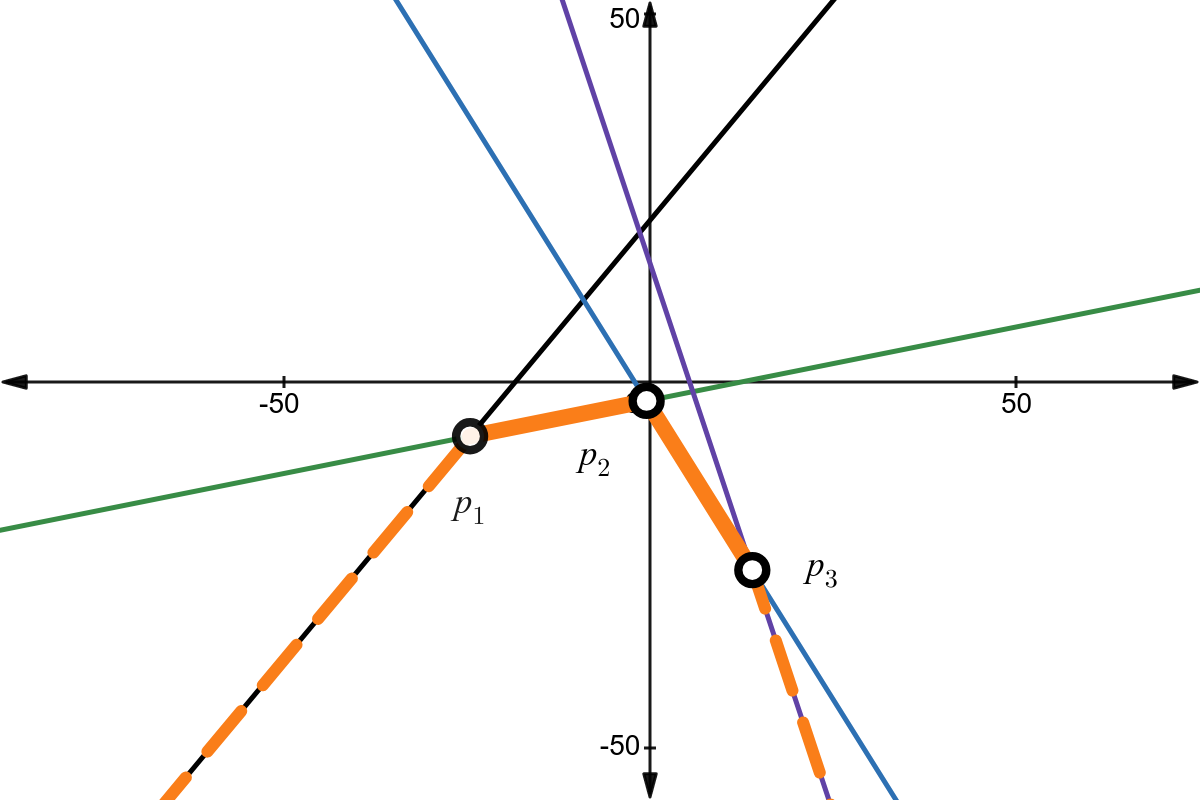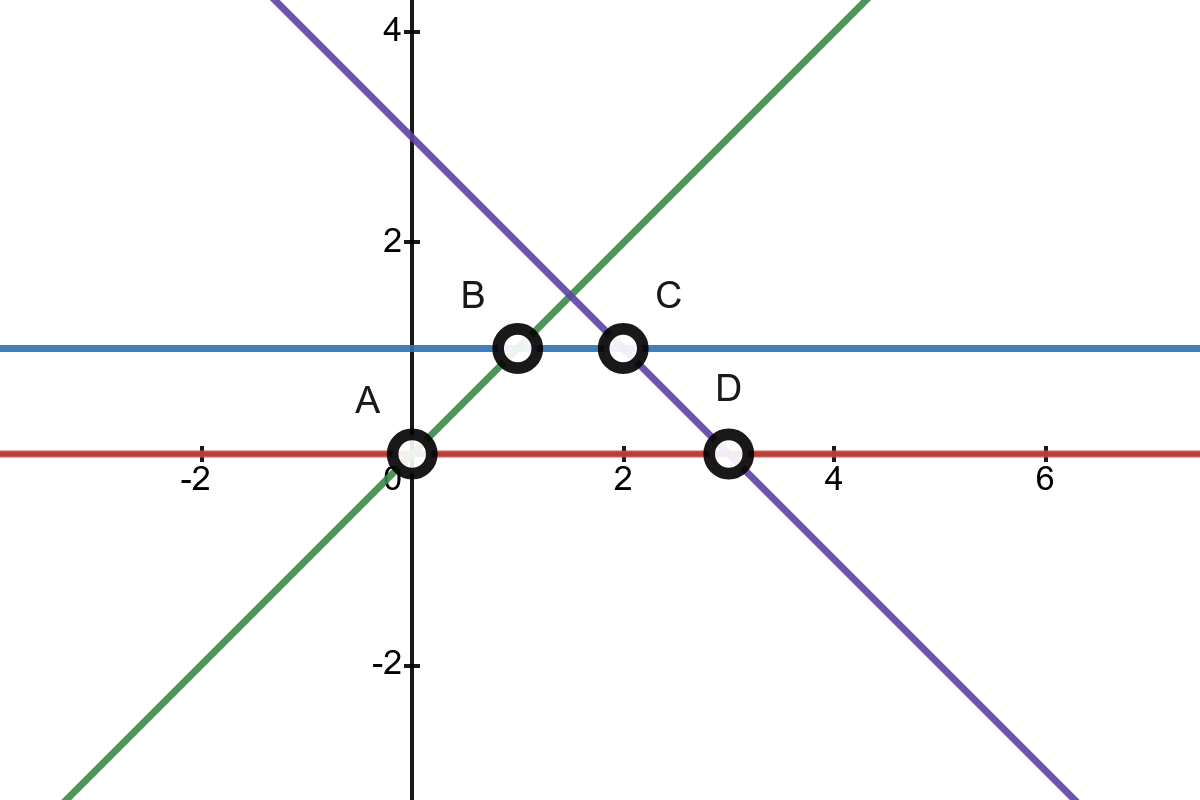## Lower envelope

View as PDF

Points: 1.60 (partial)
Time limit: 2.0s
Memory limit: 256M
Input: stdin
Output: stdout

Problem type
Allowed languages
C, C++, Java, Kotlin, Pascal, PyPy, Python, Scratch

The lower envelope of a set of ~n~ lines, in which the ~i~-th line has the equation ~y_i = a_i \cdot x + b_i~, has the graphical representation ~y = \min_{i = 1}^n\{ a_i \cdot x + b_i \}~.

We call the vertices of a lower envelope the points that lie on the lower envelope that are also the intersection of two different segments in the set. Let us suppose that the lower envelope has ~k~ vertices ~p_1, p_2, \ldots, p_k~, with vertex ~p_i~ at a smaller x-coordinate than ~p_{i + 1}~ (~1 \le i < k~). We call the perimeter of the lower envelope being the sum of distances between every two consecutive vertices of the lower envelope, formally ~p_1 p_2 + p_2 p_3 + \ldots p_{k - 1} p_k~. If the lower envelope contains less than two vertices, we consider the perimeter to be ~0~.The lower envelope is colored orange. It has three vertices ~p_1~, ~p_2~ and ~p_3~. The perimeter is denoted by the continuous orange segment.

You are given ~n~ lines. For each line, find the perimeter of the lower envelope created by the remaining ~n - 1~ lines.

#### Input

The first line contains an integer ~n~ (~2 \le n \le 100\, 000~), the number of lines.

The ~i~-th out of the next ~n~ lines contains two integers ~a_i~ và ~b_i~ (~|a_i|, |b_i| \le 10^9~), the parameters of the ~i~-th line.

It is guaranteed that there are no two lines that are the same.

#### Output

Output ~n~ lines. The ~i~-th of which contains the perimeter of the lower envelope made by the other ~n - 1~ lines, that is, excluding line ~i~.

Your answer is considered correct if its absolute or relative error does not exceed ~10^{-6}~.

#### Scoring

• Subtask 1 (~30~ points): ~n \le 100~.

• Subtask 2 (~40~ points): ~n \le 1000~.

The total score for this problem is ~200~.

#### Sample Input 1

4
0 0
0 1
1 0
-1 3


#### Sample Output 1

1.0
3.0
0
0


#### Sample Input 2

5
4 21
-3 -13
1 -3
3 15
1 -1


#### Sample Output 2

9.1923881554
0.0000000000
6.1282587703
7.7781745931
7.7781745931


#### Notes

Below is the illustration for sample 1.• The first line has the equation ~y = 0x + 0~ colored red. Excluding this line, the lower envelope has vertices ~B~ và ~C~.

• The second line has the equation ~y = 0x + 1~ colored blue. Excluding this line, the lower envelope has vertices ~A~ và ~D~.

• The third line has the equation ~y = x~ colored green. Excluding this line, the lower envelope has vertices ~D~.

• The fourth line has the equation ~y = -x + 3~ colored purple. Excluding this line, the lower envelope has vertices ~A~.